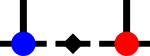SyTen## ◆ GenericDenseTensor

template<Rank rank, typename Scalar = SDef>
 using syten::GenericDenseTensor = typedef GenericDenseTensorImpl::GenericDenseTensor

Generic dense tensor class.

The generic dense tensor is a variant of specialised dense tensor classes. Some functions are already defined on it (like GenericDenseTensor::dim(), GenericDenseTensor::dims() or GenericDenseTensor::size()) but for most operations, you first need to obtain a reference to the desired stored dense tensor. Typically, this leads to structure like

auto const& x = block.m.standard();
Scalar acc = 0.;
for(Size i = 0; i != x.size(); ++i) {
acc += x[i];
}

In particular, one needs to ensure that .standard() (or similar calls) are made outside inner loops, as we otherwise infer a large overhead.

If only standard dense tensors are supported at a particular location, the above code is fully sufficient and will either fail loudly (with an exception) if a non-standard dense tensor is used or work efficiently. To transform a non-standard dense tensor into a dense tensor, use GenericDenseTensor::make_standard(), to make a Cuda tensor likewise use GenericDenseTensor::make_cuda() (and GenericDenseTensor::maybe_make_cuda() to do so only if advantageous).

Currently implemented specialised dense tensor classes are syten::DenseTensor, syten::OffsetDenseTensor, syten::IdentityDenseTensor and syten::CudaDenseTensor.

Each of these classes needs to support a minimal interface consisting of the following functions:

• .dim(Index) const returning the dimension of the 1-indexed leg
• .dims() const returning an array of dimensions
• .size() const returning the number of stored dense entries
• normSqd(XDenseTensor const&) returning the squared norm
• isZero(XDenseTensor const&) returning true only if all elements of the tensor are identically zero.
• explicit .operator DenseTensor() const: explicit conversion operator to a standard dense tensor.

Additional operators may be implemented as appropriate.